# Seminar 5

In this seminar, we will use Y.RData file that we created in the Seminar 2. We focus on coding multivariate volatility models using rmgarch package. Please review the theory of bivariate EWMA and DCC models that we discussed in the lecture.

The plan for this week:

The following sections and subsections are materials for students to learn by themselves: DCC apARCH and tapARCH and DCC with several assets.

We first load the packages for this seminar:

In :
library(rmgarch)
library(lubridate)


## Multivariate volatility models¶

In a univariate volatility model, we consider a stock with returns $Y_t$ that can be written as:

$$Y_t = \sigma_{t} Z_{t}$$

Where $\sigma_t$ is the conditional volatility and $Z_t$ are random shocks.

For many financial applications, we have to consider a vector of assets, instead of a single asset. So, if we have $K>1$ assets, it is necessary to indeicate which asset and paremeters are being referred to. The notation we will use is:

$$Y_{t,i} = \sigma_{t,i} Z_{t,i}$$

Where the first subscript indicates the date, and the second subscript the asset.

Now our conditional covariance is a symmetric square conditional covariance matrix, where the dimension is the number of assets. For example, for three assets it would be:

$$\begin{equation*} \Sigma_{t} = \begin{pmatrix} \sigma_{t,11} & & \\ \sigma_{t,12} & \sigma_{t,22} & \\ \sigma_{t,13} & \sigma_{t,23} & \sigma_{t,33} \end{pmatrix} \end{equation*}$$

If we have a portfolio wight a vector $w$ of portfolio weights, then the portfolio variance would be:

$$\sigma^2_{\textrm{portfolio}} = w'\Sigma w$$

As in the case of univariate models, we need to ensure that the variance is not negative. In this case, this means that the covariance matrix $\Sigma$ is positive semi-definite:

$$|\Sigma| \geq 0$$

Working with several assets presents the curse of dimensionality. This means that the number of variance and covariance terms will explode as the number of assets increase, which makes it challenging to estimate the covariance matrix and ensure its positive semi-definiteness.

For example, if we try to estimate a GARCH model for two assets, we would have 21 parameters to estimate, which is almost impossible in practice.

The concept of stationarity is more important in multivariate volatility models, since violating it could lead to numerical problems. In this seminar we will focus in implementing two different multivariate models:

• Exponentially-Weighted Moving Average
• Dynamic Conditional Correlation Models

To do so, we will work with the returns from JP Morgan and Citigroup.

In :
# Load the data

# Extract the returns for JPM and C
y <- Y[c("JPM", "C")]


## EWMA¶

In the Exponentially-Weighted Moving Average model, the estimated conditional volatility at time $t$ is a convex combination of the estimation at time $t-1$ and the squared returns at time $t-1$. In general, if we have a vector of returns for each time $t$ that includes all assets $K$:

$$\textbf{y}_{t} = [y_{t,1}, y_{t,2}, \ldots, y_{t,K}]$$

Then the multivariate EWMA is:

$$\hat{\Sigma}_{t} = \lambda \hat{\Sigma}_{t-1} + (1-\lambda) \textbf{y}'_{t-1} \textbf{y}_{t-1}$$

The properties of this mdoel are:

• The same pre-specified weight, $\lambda$ is used for all assets
• The variance of any particular asset only depends on its own lags

### Implementation¶

Since we will perform linear algebra operations to compute the EWMA, it is more convenient to work with a matrix instead of a dataframe, so let's first turn y from a dataframe into a matrix:

In :
# Check the class of y
class(y)

# Transform into matrix
y  <- as.matrix(y)

# Check class again
class(y)

'data.frame'
1. 'matrix'
2. 'array'

Let's take a look at the returns:

In :
# Plotting the returns in a 2x1 grid
par(mfrow = c(2,1))
plot(y[,1], type = "l", main = "Returns for JPM", col = 1)
plot(y[,2], type = "l", main = "Returns for C", col = 2)
# Reset the grid
par(mfrow = c(1,1))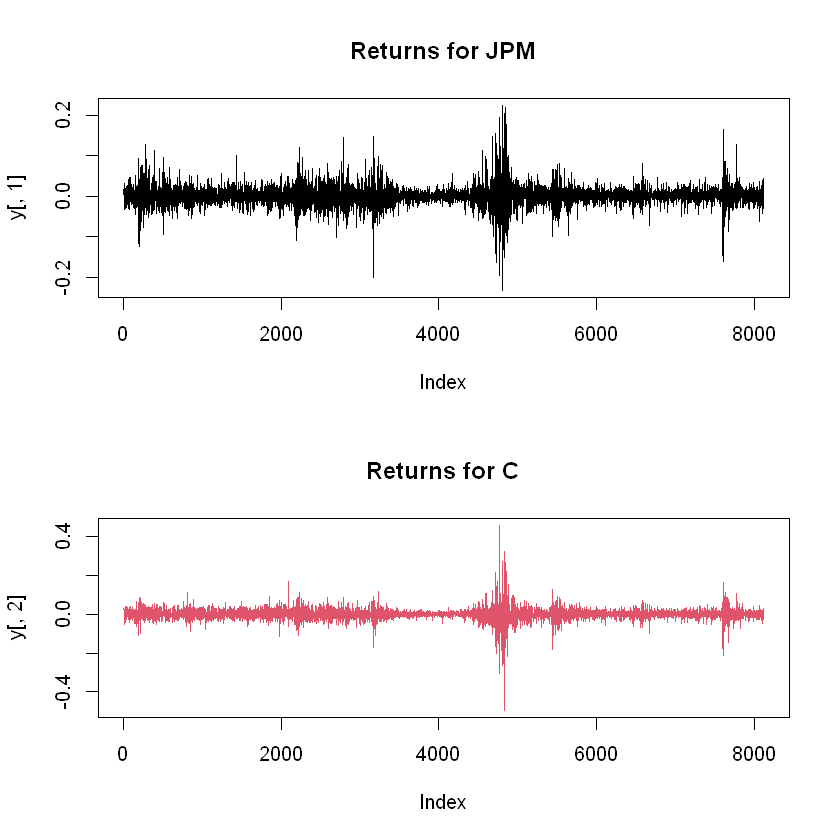We will create a EWMA matrix that will hold our estimations for the elements of $\Sigma$. First let's establish the number of entries the matrix should have:

In :
# Determine number of entries
n <- dim(y)


Now we create the matrix. We could initialize the matrix with a number, like 0, but it is recommended to initialize it with NA to avoid any potential mistakes.

To determine the number of columns in the matrix, we need to figure out how many parameters we are estimating in every time period. For any given time $t$, we will be estimating the conditional variance of each asset, and the conditional covariances. For $K$ assets, this is:

$$K + K(K-1)/2$$

In our case, it is 3. Column 1 will hold the conditional variance of JPM, column 2 will hold the conditional covariance between JPM and C, and column 3 will hold the conditional variance for Citigroup:

In :
# Initializing the EWMA matrix
EWMA <- matrix(NA, nrow = n, ncol = 3)


#### Why do we initialize a matrix/vector?¶

It is convenient to create the vector or matrix we want to populate before doing so for two reasons:

1. Good programming practice. It is better to have a fixed-length object than to re-size an object in every iteration of the loop.
2. Be able to spot mistakes. In case there is an error in your loop and try to allocate an element to a non-existing row, you will get an error message. If you are dynamically modifying the size of the object, you can add more rows/columns by accident without realizing it

We could fill the new matrix with any values, and it is common to fill them with zeros. However, it is useful to fill the matrix with NA. This way, if something in the loop doesn't work, our matrix will have some empty spots instead of holding zeros, and whenever we try to make any operation we will get an error message.

We determine the value for $\lambda$:

In :
# Determine lambda
lambda <- 0.94


It is necessary is to determine how to estimate the conditional covariances of the first period. In other words, how do we estimate $\hat{\Sigma}_1$ without $\hat{\Sigma}_0$ or $\textbf{y}_0$. For this, we will use the unconditional covariance of the sample and "burn" the first few observations. The effect of a given conditional covariance from a past period quickly dies out as time passes, because $\lambda^n \rightarrow 0$ as $n \rightarrow \infty$, so the effect of initializing the EWMA matrix with the unconditional covariance will not be a problem after a few time periods. A rule of thumb is to burn the first 30 observations.

In :
# Get the sample covariance
S <- cov(y)
S

A matrix: 2 × 2 of type dbl
JPMC
JPM0.00055557090.0004534512
C0.00045345120.0007880355

We want to get the three unique values of the matrix. This can be done in three different ways shown below. We recommend using the last, since it is easily reproducible for any number of assets:

In :
# Getting the unique values in three ways:

# 1. Creating a vector with the distinct elements
c(S[1,1], S[1,2], S[2,2])

# 2. Vectorizing the matrix and getting distinct elements
c(S)[c(1,2,4)]

# 3. Using the fact that S is symmetric and using upper.tri()/lower.tri()
# upper.tri() returns a matrix of logicals
# with entries TRUE in the upper triangle
S[upper.tri(S, diag = TRUE)]

1. 0.000555570942953469
2. 0.00045345122972662
3. 0.00078803548191968
1. 0.000555570942953469
2. 0.00045345122972662
3. 0.00078803548191968
1. 0.000555570942953469
2. 0.00045345122972662
3. 0.00078803548191968
In :
# Fill the initial row of EWMA with the sample covariances
EWMA[1,] <- S[upper.tri(S, diag = TRUE)]

# View the matrix

 0.0005555709 0.0004534512 0.0007880355 NA NA NA NA NA NA NA NA NA NA NA NA NA NA NA

The first column is the sample variance of JPM, the second column is the sample covariance, and the third column is the sample variance of C.

As an example, let's manually compute how we would estimate the variances and covariance for $t=2$:

In :
# Manually computing EWMA elements for t = 2

# Apply the formula for EWMA
S_2 <- lambda * S + (1-lambda) * y[1,] %*% t(y[1,])
# Get the variances and covariances
S_2[upper.tri(S_2, diag = TRUE)]

1. 0.000523282659857329
2. 0.000433819799325835
3. 0.00079562125711708

Now that we have seen how to get the elements for a given time, we can write a for loop to populate the entire EWMA matrix:

In :
# Populating the EWMA matrix

# Create a loop for rows 2 to n
for (i in 2:n) {
# Update S with the new weighted moving average
S <- lambda * S + (1-lambda) * y[i-1,] %*% t(y[i-1,])

# Fill the following EWMA row with the covariances
EWMA[i,] <- S[upper.tri(S, diag = TRUE)]
}


Let's see the head() of our EWMA matrix:

In :
head(EWMA)

 0.000555571 0.000453451 0.000788036 0.000523283 0.00043382 0.000795621 0.000556395 0.000432747 0.000757539 0.000523983 0.00040372 0.000721741 0.000493507 0.000381535 0.000682746 0.000463897 0.000358642 0.000646019

### Important note on Matrix Operations¶

Since we are working with matrices, it is very important to pay attention to the dimensions of the elements, to make sure we don't do any mistake when multiplying vectors. Here is a short review on the order of vectors for multiplication:

In :
# Matrix operations - The order is important

# This is a 2x1 vector
y[1,]

# This is a scalar (a 1x2 row vector multiples a 2x1 column vector)
t(y[1,]) %*% y[1,]

# This is a matrix (a 2x1 column vector multiples a 1x2 row vector)
y[1,] %*% t(y[1,])

JPM
0.0041752714104756
C
0.0302401234875621
 0.000931898
A matrix: 2 × 2 of type dbl
JPMC
1.743289e-050.0001262607
1.262607e-040.0009144651

Let's create a wrong_EWMA matrix to show what would happen if we were to mix up the transposes:

In :
# Creating a wrong EWMA

# Initialize the matrix the same way
wrong_EWMA <- matrix(NA, nrow = n, ncol = 3)
S <- cov(y)
wrong_EWMA[1,] <- S[upper.tri(S, diag = TRUE)]

# Do the loop but interchange the transpose
for (i in 2:n) {
# Update S with the new weighted moving average
S <- lambda * S + (1-lambda) * t(y[i-1,]) %*% y[i-1,]

# Fill the following EWMA row with the covariances
wrong_EWMA[i,] <- S[upper.tri(S, diag = TRUE)]
}

Error in lambda * S + (1 - lambda) * t(y[i - 1, ]) %*% y[i - 1, ]: non-conformable arrays
Traceback:


### Plotting the conditional variances and covariances¶

In :
# Plotting estimated variances and covariances
matplot(EWMA, type = "l", main = "EWMA", lty = 1)
legend("topleft", legend = c("JPM", "Covar", "C"), col = 1:3, lty = 1)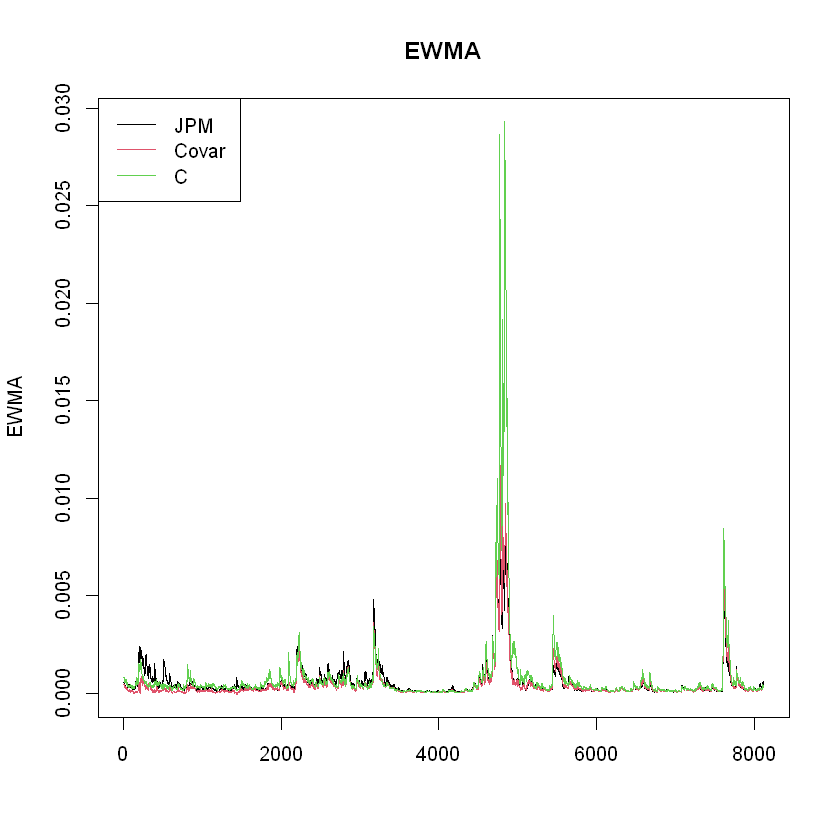We can calculate the correlation coefficient of the two stocks, which is the covariance over the square root of variances:

In :
# Correlation coefficient
EWMArho <- EWMA[,2]/sqrt(EWMA[,1]*EWMA[,3])

# Plot
plot(EWMArho, type = "l", main = "Correlation coefficient of JPM and C", col = "red")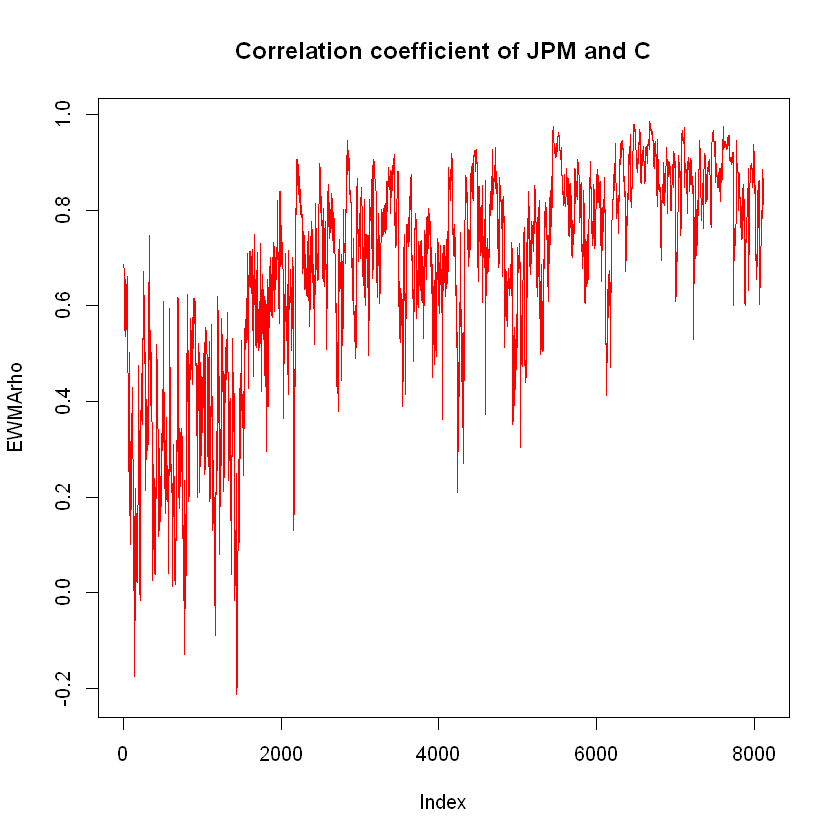Let's replicate the plots including the date as the x-axis. We can get it from our Y data frame:

In :
# Plots for conditional volatility
plot(Y$date, sqrt(EWMA[,1]), type = "l", main = "Conditional volatility JPM") plot(Y$date, sqrt(EWMA[,3]), type = "l", main = "Conditional volatility C")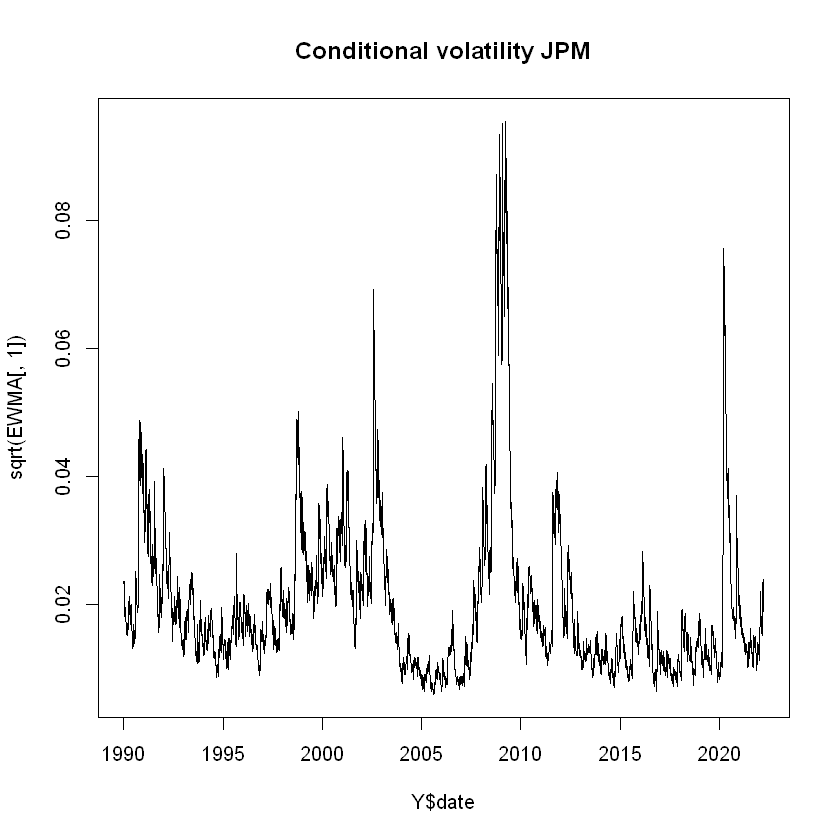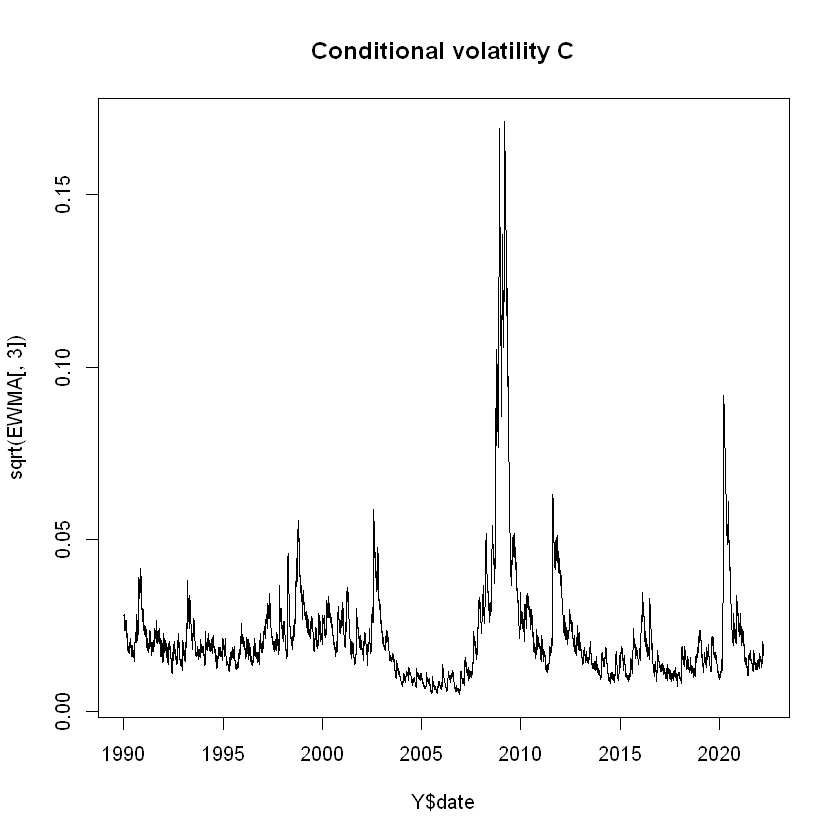In :
# Correlation Coefficient plot
plot(Y$date, EWMArho, type = "l", main = "Correlation coefficient of JPM and C", col = "red")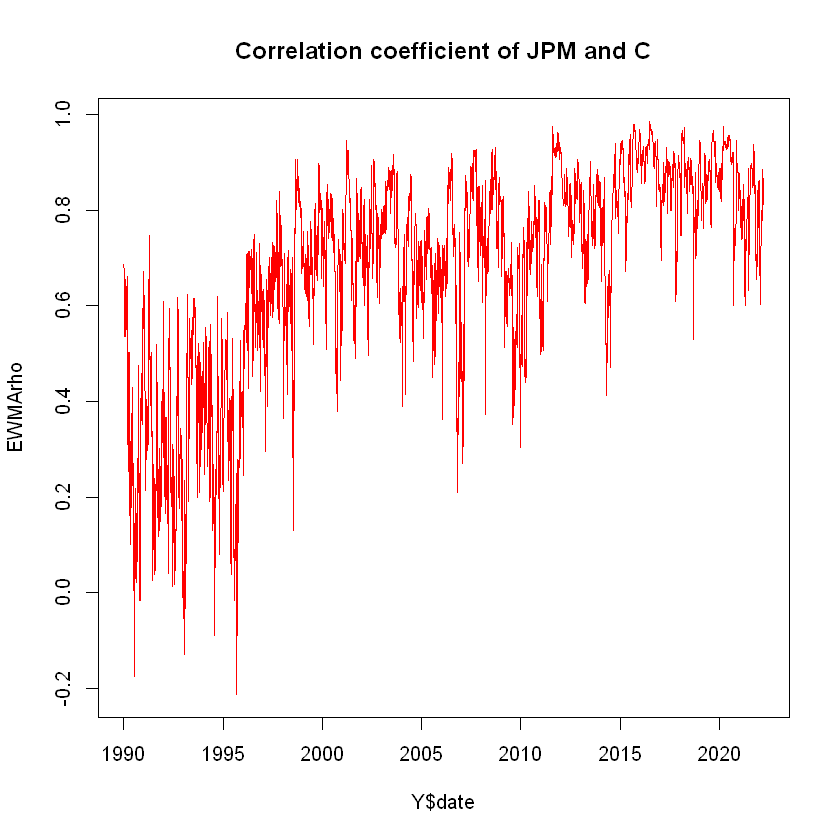## Dynamic Conditional Correlation models¶ To discuss and implement Dynamic Conditional Correlation (DCC) models, we first have to discuss Constant Conditional Correlation (CCC) models. In this type of model, we separate out correlation modeling from volatility model. For the former, we use a correlation matrix, and for the latter we use GARCH or some standard method. We have discussed the theory of DCC models in lecture. We will implement the DCC model using the rmgarch package, which is the multivariate version of rugarch. ### Implementation¶ Similarly to a univariate model from rugarch, we need to specify the model we are going to use. In this case, we use the dccspec() function. We will replicate a univariate specification for each asset and implement both in the DCC model: In : # DCC model # Specify the default univariate GARCH model with no mean xspec <- ugarchspec( mean.model = list(armaOrder = c(0,0), include.mean = FALSE) ) # Replicate it into a multispec() element uspec <- multispec(replicate(2, xspec)) # Define the specification for the DCC model spec <- dccspec( # GARCH specification uspec = uspec, # DCC specification dccOrder = c(1,1), # Distribution, here multivariate normal distribution = "mvnorm" ) # Fit the specification to the data res <- dccfit(spec, data = y)  We can call the variable to see the fitted model: In : # Call the variable res  *---------------------------------* * DCC GARCH Fit * *---------------------------------* Distribution : mvnorm Model : DCC(1,1) No. Parameters : 9 [VAR GARCH DCC UncQ] : [0+6+2+1] No. Series : 2 No. Obs. : 8126 Log-Likelihood : 44508.91 Av.Log-Likelihood : 5.48 Optimal Parameters ----------------------------------- Estimate Std. Error t value Pr(>|t|) [JPM].omega 0.000003 0.000006 0.524024 0.600262 [JPM].alpha1 0.076259 0.044481 1.714411 0.086453 [JPM].beta1 0.919049 0.047694 19.269574 0.000000 [C].omega 0.000003 0.000041 0.061506 0.950956 [C].alpha1 0.075481 0.312946 0.241196 0.809403 [C].beta1 0.923325 0.316322 2.918938 0.003512 [Joint]dcca1 0.025158 0.003653 6.885988 0.000000 [Joint]dccb1 0.972668 0.004501 216.118979 0.000000 Information Criteria --------------------- Akaike -10.952 Bayes -10.945 Shibata -10.952 Hannan-Quinn -10.950 Elapsed time : 4.501322  The output includes the GARCH parameters$\alpha$and$\beta$for each one of the assets, and the joint DCC parameters$\zeta$and$\xi$respectively. In : coef(res)  [JPM].omega 3.12318841360853e-06 [JPM].alpha1 0.0762591600110328 [JPM].beta1 0.919048703948558 [C].omega 2.50599356676793e-06 [C].alpha1 0.0754813014288934 [C].beta1 0.923325384800986 [Joint]dcca1 0.025157550799261 [Joint]dccb1 0.972667557806248 ### Using plot( ) on a DCC object¶ Calling the function plot() on a DCC object gives us a menu of options: Make a plot selection (or 0 to exit): 1. Conditional Mean (vs Realized Returns) 2. Conditional Sigma (vs Realized Absolute Returns) 3. Conditional Covariance 4. Conditional Correlation 5. EW Portfolio Plot with conditional density VaR limits  Some of these plots, like Plot 2, 3 and 4, include information similar to what we plotted in the case of EWMA (The plot is different for different time span): #### Plot 2¶#### Plot 3¶#### Plot 4¶### Exploring a DCC object¶ We can use the @ operator on the DCC object to explore what output is provided: In : # Explore DCC object names(res@mfit)  1. 'coef' 2. 'matcoef' 3. 'garchnames' 4. 'dccnames' 5. 'cvar' 6. 'scores' 7. 'R' 8. 'H' 9. 'Q' 10. 'stdresid' 11. 'llh' 12. 'log.likelihoods' 13. 'timer' 14. 'convergence' 15. 'Nbar' 16. 'Qbar' 17. 'plik' Let's extract the H matrix, which includes the covariances: In : # Extracting the H matrix H <- res@mfit$H

# Dimensions of the H matrix
dim(H)

1. 2
2. 2
3. 8126

The H matrix has three dimensions. You can think of it as a set that contains 8126 2x2 matrices. Each 2x2 matrix is a variance-covariance matrix, and we have one for each time period. We can access it the same way as we would access a two-dimensional matrix, but adding one more element within the square brackets.

If we want to see the first period's matrix:

In :
# First period's covariances
H[,,1]

 0.000555705 0.00044835 0.00044835 0.000787971

Let's see the first four covariance matrix estimations:

In :
# First four covariance matrix estimations
print(H[,,1:4])

, , 1

[,1]         [,2]
[1,] 0.0005557046 0.0004483496
[2,] 0.0004483496 0.0007879706

, , 2

[,1]         [,2]
[1,] 0.0005151722 0.0004312821
[2,] 0.0004312821 0.0007990843

, , 3

[,1]         [,2]
[1,] 0.0005585817 0.0004337752
[2,] 0.0004337752 0.0007524668

, , 4

[,1]         [,2]
[1,] 0.0005177217 0.0004029693
[2,] 0.0004029693 0.0007094236



If we want to compute the conditional correlations of the DCC model, we can extract the variances and covariances from the H matrix. Recall the covariance matrix is a symmetric matrix and the variances are found in the diagonal of the matrix while the covariance will be found in the off-diagonal element:

In :
# Computing the conditional correlations

# Initializing the vector
rhoDCC <- vector(length = n)

# Populate with the correlations
rhoDCC <- H[1,2,] / sqrt(H[1,1,]*H[2,2,])


### DCC apARCH and tapARCH (Not covered in class)¶

Let's fit more DCC models using different univariate specification for the estimations of the assets' returns.

First, consider a DCC apARCH:

In :
# DCC apARCH model

# Univariate specification
xspec <- ugarchspec(variance.model = list(model = "apARCH"),
mean.model = list(armaOrder = c(0,0), include.mean = FALSE)
)

# Duplicate the specification using multispec
uspec <- multispec(replicate(2, xspec))

# Create the DCC specification
spec <- dccspec(uspec = uspec,
dccOrder = c(1,1),
distribution = "mvnorm")

# Fit it to the data
res_aparch <- dccfit(spec, data = y)

# Call the object
res_aparch

*---------------------------------*
*          DCC GARCH Fit          *
*---------------------------------*

Distribution         :  mvnorm
Model                :  DCC(1,1)
No. Parameters       :  13
[VAR GARCH DCC UncQ] : [0+10+2+1]
No. Series           :  2
No. Obs.             :  8126
Log-Likelihood       :  44609.19
Av.Log-Likelihood    :  5.49

Optimal Parameters
-----------------------------------
Estimate  Std. Error   t value Pr(>|t|)
[JPM].omega   0.000066    0.000106   0.62085 0.534699
[JPM].alpha1  0.073196    0.062168   1.17739 0.239038
[JPM].beta1   0.930714    0.069932  13.30887 0.000000
[JPM].gamma1  0.470010    0.360047   1.30541 0.191753
[JPM].delta   1.295359    0.666791   1.94268 0.052055
[C].omega     0.000108    0.001056   0.10278 0.918142
[C].alpha1    0.080033    0.395532   0.20234 0.839648
[C].beta1     0.930000    0.401739   2.31494 0.020617
[C].gamma1    0.403163    2.076161   0.19419 0.846030
[C].delta     1.143207    4.191174   0.27276 0.785034
[Joint]dcca1  0.027181    0.003403   7.98810 0.000000
[Joint]dccb1  0.970500    0.004189 231.70522 0.000000

Information Criteria
---------------------

Akaike       -10.976
Bayes        -10.965
Shibata      -10.976
Hannan-Quinn -10.972

Elapsed time : 9.177771


The output of the DCC apARCH includes the extra apARCH parameters for each individual stock. We can enrich this model further by using a multivariate T distribution as the joint distribution between the returns instead of a multivariate normal:

In :
# DCC tapARCH model

# Univariate specification
xspec <- ugarchspec(variance.model = list(model = "apARCH"),
mean.model = list(armaOrder = c(0,0), include.mean = FALSE)
)

# Duplicate the specification using multispec
uspec <- multispec(replicate(2, xspec))

# Create the DCC specification, replace the multivariate normal by a multivariate T
spec <- dccspec(uspec = uspec,
dccOrder = c(1,1),
distribution = "mvt")

# Fit it to the data
res_taparch <- dccfit(spec, data = y)

# Call the object
res_taparch

*---------------------------------*
*          DCC GARCH Fit          *
*---------------------------------*

Distribution         :  mvt
Model                :  DCC(1,1)
No. Parameters       :  14
[VAR GARCH DCC UncQ] : [0+10+3+1]
No. Series           :  2
No. Obs.             :  8126
Log-Likelihood       :  45226.77
Av.Log-Likelihood    :  5.57

Optimal Parameters
-----------------------------------
Estimate  Std. Error   t value Pr(>|t|)
[JPM].omega    0.000066    0.000106   0.62267 0.533500
[JPM].alpha1   0.073196    0.062028   1.18006 0.237975
[JPM].beta1    0.930714    0.069764  13.34086 0.000000
[JPM].gamma1   0.470010    0.359370   1.30787 0.190917
[JPM].delta    1.295359    0.664770   1.94858 0.051345
[C].omega      0.000108    0.001053   0.10299 0.917972
[C].alpha1     0.080033    0.394835   0.20270 0.839369
[C].beta1      0.930000    0.401036   2.31900 0.020395
[C].gamma1     0.403163    2.072352   0.19454 0.845750
[C].delta      1.143207    4.183071   0.27329 0.784627
[Joint]dcca1   0.028116    0.004220   6.66198 0.000000
[Joint]dccb1   0.971050    0.004571 212.41771 0.000000
[Joint]mshape  6.055768    0.213760  28.32974 0.000000

Information Criteria
---------------------

Akaike       -11.128
Bayes        -11.116
Shibata      -11.128
Hannan-Quinn -11.124

Elapsed time : 9.565642


Each stock has five individual parameters, three that are part of the standard GARCH estimation (omega, alpha, beta), and two that are particular to the apARCH model (gamma and delta). Additionally, the joint parameters include mshape, which is the estimation for the degrees of freedom of the multivariate T distribution.

Let's extract the estimated variances and covariances to create the conditional correlations vector:

In :
# Creating the conditional correlations vector from the tapARCH model

# Extracting covariances
H <- res_taparch@mfit$H # Initializing vector rhoDCCRich <- vector(length = n) # Fill the covariance matri rhoDCCRich <- H[1,2,] / sqrt(H[1,1,] * H[2,2,])  ### DCC with several assets (Not covered in class)¶ Now instead of using two assets, let's build a DCC model using all the assets in our Y data frame: In : # DCC model for all assets # Extracting all the columns from Y except the date y_all <- subset(Y, select = -c(date, int_date)) # See y head(y_all)  A data.frame: 6 × 6 MSFTXOMGEJPMINTCC <dbl><dbl><dbl><dbl><dbl><dbl> 1 0.019915366 0.00000000 0.034289343 0.004175271 0.042559364 0.030240123 2 0.005618188-0.01005034-0.001874756 0.032789500-0.028171106 0.012685202 3 0.028987765-0.01015236-0.005644903 0.004023893 0.021202627-0.012685117 4-0.024794868-0.00511506-0.009478782 0.004007957-0.007017566 0.008474986 5 0.015225502 0.01526785 0.005697737 0.000000000 0.013986728 0.008403591 6-0.002750780-0.02040885-0.021053069-0.032523192 0.027399190-0.012631442 In : # Transform to matrix y_all <- as.matrix(y_all) # Check dimensions dim(y_all)  1. 8126 2. 6 In : # Build DCC model # Univariate spec xspec <- ugarchspec(mean.model = list(armaOrder = c(0,0), include.mean = FALSE)) # Replicate for each asset uspec <- multispec(replicate(dim(y_all), xspec)) # Build DCC spec spec <- dccspec(uspec = uspec, dccOrder = c(1,1), distribution = "mvnorm") # Fit the model dcc_all <- dccfit(spec, data = y_all) # Call the object dcc_all  *---------------------------------* * DCC GARCH Fit * *---------------------------------* Distribution : mvnorm Model : DCC(1,1) No. Parameters : 35 [VAR GARCH DCC UncQ] : [0+18+2+15] No. Series : 6 No. Obs. : 8126 Log-Likelihood : 136554 Av.Log-Likelihood : 16.8 Optimal Parameters ----------------------------------- Estimate Std. Error t value Pr(>|t|) [MSFT].omega 0.000006 0.005339 1.1300e-03 0.999099 [MSFT].alpha1 0.072585 1.016859 7.1381e-02 0.943094 [MSFT].beta1 0.913579 18.375487 4.9717e-02 0.960348 [XOM].omega 0.000003 0.000006 4.4157e-01 0.658802 [XOM].alpha1 0.071618 0.050488 1.4185e+00 0.156041 [XOM].beta1 0.917692 0.057769 1.5885e+01 0.000000 [GE].omega 0.000001 0.000001 1.0076e+00 0.313653 [GE].alpha1 0.057706 0.011517 5.0106e+00 0.000001 [GE].beta1 0.939488 0.011870 7.9149e+01 0.000000 [JPM].omega 0.000003 0.000006 5.2465e-01 0.599825 [JPM].alpha1 0.076259 0.044424 1.7166e+00 0.086047 [JPM].beta1 0.919049 0.047637 1.9293e+01 0.000000 [INTC].omega 0.000003 0.000001 3.1303e+00 0.001746 [INTC].alpha1 0.032704 0.001201 2.7237e+01 0.000000 [INTC].beta1 0.962996 0.000500 1.9255e+03 0.000000 [C].omega 0.000003 0.000041 6.1739e-02 0.950771 [C].alpha1 0.075481 0.311762 2.4211e-01 0.808693 [C].beta1 0.923325 0.315145 2.9298e+00 0.003391 [Joint]dcca1 0.009889 0.005959 1.6596e+00 0.097003 [Joint]dccb1 0.987203 0.008023 1.2304e+02 0.000000 Information Criteria --------------------- Akaike -33.601 Bayes -33.570 Shibata -33.601 Hannan-Quinn -33.590 Elapsed time : 12.08615  Each stock has three parameters, and there are two joint ones. In total we have 20 parameters. Let's now extract the H matrix and see how it looks: In : # Extracting H matrix H <- dcc_all@mfit$H

# Check the dimensions
dim(H)

# See the first one - 6x6 matrix
H[,,1]

1. 6
2. 6
3. 8126
 0.000400638 8.81917e-05 0.00013916 0.00017324 0.000249091 0.000205103 8.81917e-05 0.000242423 0.000119679 0.000130952 0.000107556 0.000166689 0.00013916 0.000119679 0.000370564 0.000223327 0.000168239 0.000275586 0.00017324 0.000130952 0.000223327 0.000555705 0.000210501 0.000445006 0.000249091 0.000107556 0.000168239 0.000210501 0.00057388 0.000250097 0.000205103 0.000166689 0.000275586 0.000445006 0.000250097 0.000787971

To build the conditional correlations, we have to be careful on specifying which two assets we are interested in. We can see which asset belongs to which column by calling:

In :
# Check which asset is in which column
colnames(y_all)

1. 'MSFT'
2. 'XOM'
3. 'GE'
4. 'JPM'
5. 'INTC'
6. 'C'

If we wanted the conditional correlation between 'MSFT' and 'JPM', we would have to write this:

In :
# Conditional correlation between two stocks
r_msft_jpm <- H[1,4,]/sqrt(H[1,1,]*H[4,4,])


This can be annoying since we have to keep track of the numbers for each stock. Alternatively, we can write a short function that solves this problem:

In :
# Writing a function that prevents us from keeping track of numbers

cond_corr <- function(stock1, stock2) {

# Finds the index of each ticker in colnames
index1 <- which(colnames(y_all) == stock1)
index2 <- which(colnames(y_all) == stock2)

# Return the vector operation
return(H[index1, index2, ]/sqrt(H[index1, index1,]*H[index2, index2,]))
}

In :
# Call it on MSFT and JPM
r_msft_jpm_2 <- cond_corr("MSFT", "JPM")

In :
# Compare the two:

1. 0.367154994941753
2. 0.367027514782895
3. 0.367091564517041
4. 0.365462525050238
5. 0.360534943131423
6. 0.359562357635861
1. 0.367154994941753
2. 0.367027514782895
3. 0.367091564517041
4. 0.365462525050238
5. 0.360534943131423
6. 0.359562357635861

Now we can easily find the conditional correlation of stocks without worrying of the index. Let's get the conditional correlation for JP Morgan and Citigroup, and plot it over time:

In :
# JPM and C
r_jpm_c <- cond_corr("JPM", "C")

# Plot it
plot(Y$date, r_jpm_c, main = "Conditional correlation for JPM and C", type = "l", col = "red", las = 1)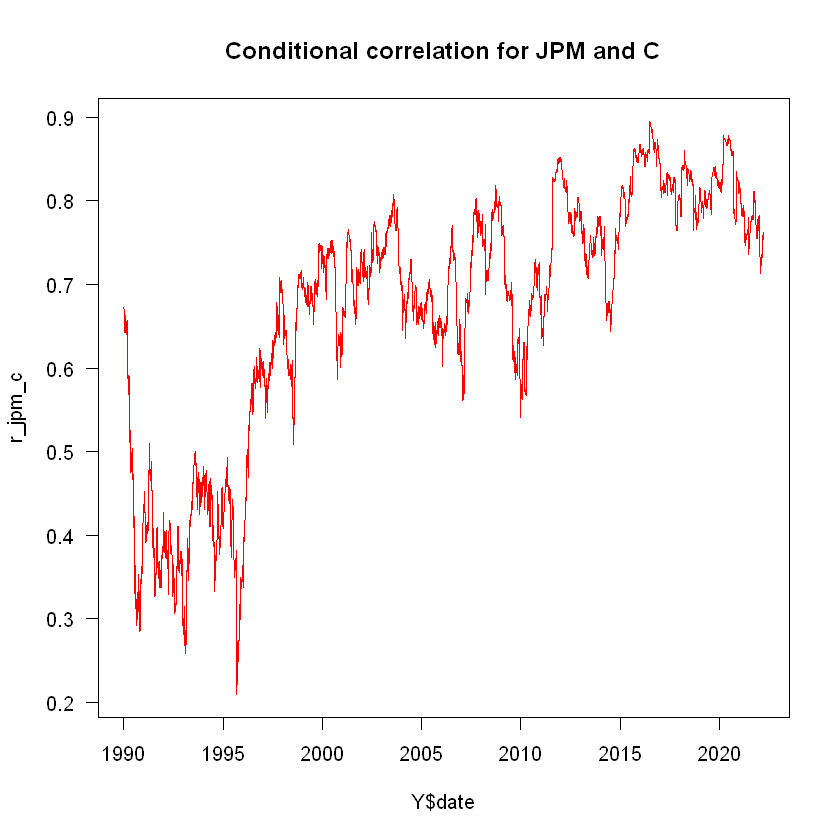Add a horizontal line at the mean: In : # Horizontal line at mean plot(Y$date, r_jpm_c, main = "Conditional correlation for JPM and C",
type = "l", col = "red", las = 1)
abline(h = mean(r_jpm_c), lwd = 2)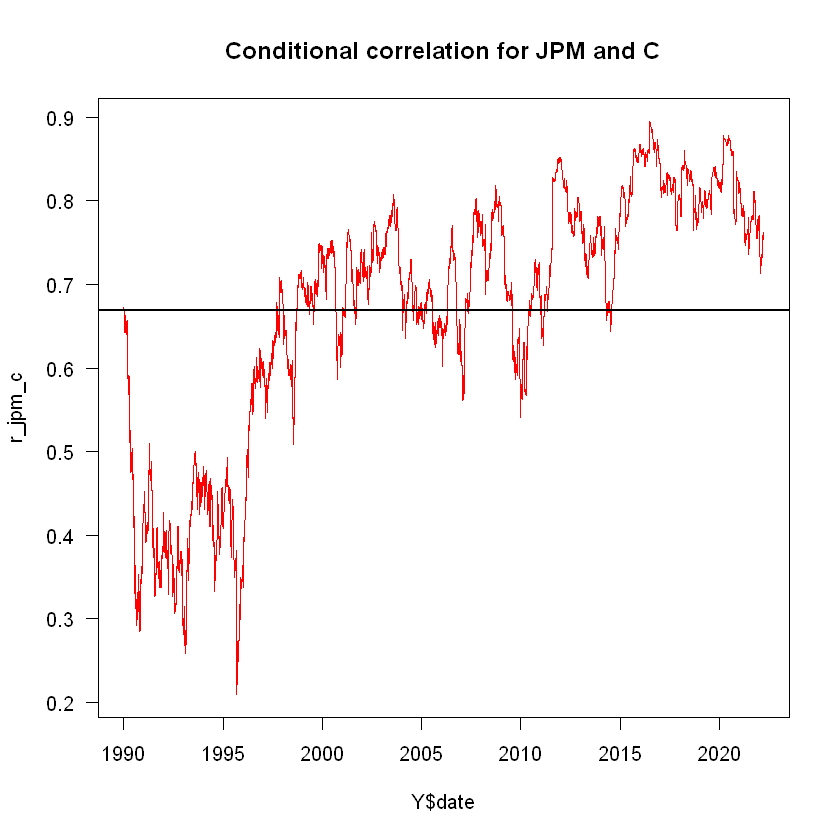## Model comparison¶

In this section we will compare both visually and numerically the output of the models we have ran. We will focus in comparing the EWMA and DCC models.

### Plotting the Conditional Correlations¶

Let's use matplot() to plot the conditional correlations obtained in EWMA, DCC, and the DCC using apARCH:

In :
# Comparing DCC and EWMA visually
matplot(cbind(EWMArho, rhoDCC, rhoDCCRich), type = "l", lty = 1,
main = "Conditional Correlations for EWMA, DCC, DCC and tapARCH")
legend("bottomright", legend = c("EWMA", "DCC", "DCC tapARCH"), lty = 1, col = 1:3)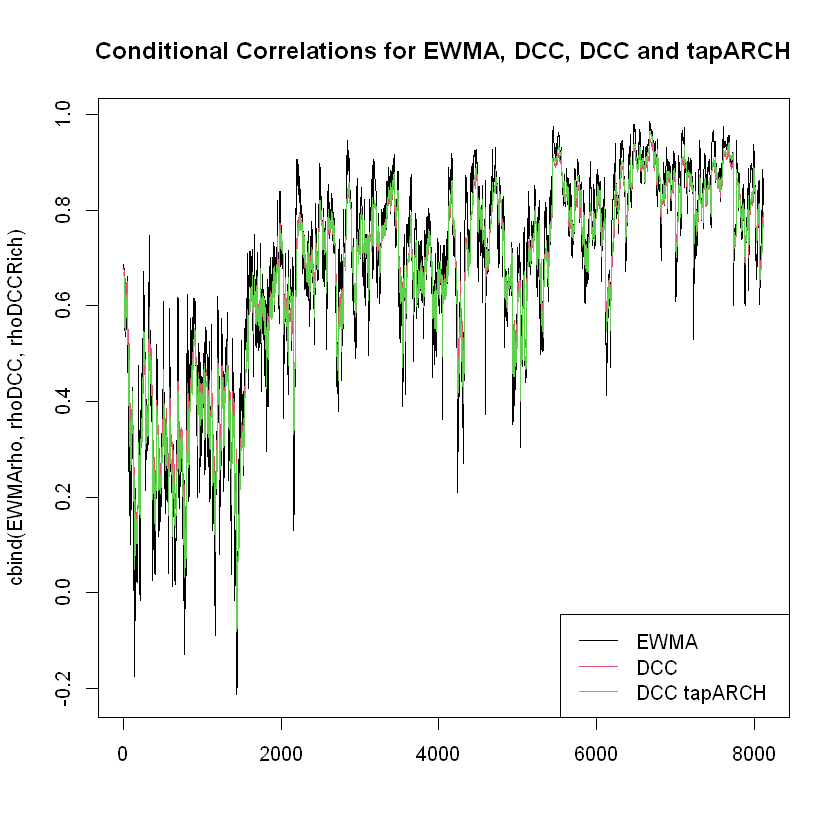We can see the estimations are quite similar. Computing the correlation between the conditional correlations of the three methods shows us a near-perfect correlation:

In :
# Correlation
cor(cbind(EWMArho, rhoDCC, rhoDCCRich))

A matrix: 3 × 3 of type dbl
EWMArhorhoDCCrhoDCCRich
EWMArho1.00000000.97168490.9745133
rhoDCC0.97168491.00000000.9982971
rhoDCCRich0.97451330.99829711.0000000

We see a strong linear relationship between EWMArho and rhoDCC:

In :
# Relationship between EWMArho and rhoDCC
plot(rhoDCC, EWMArho, main = "Relationship between EWMArho and rhoDCC")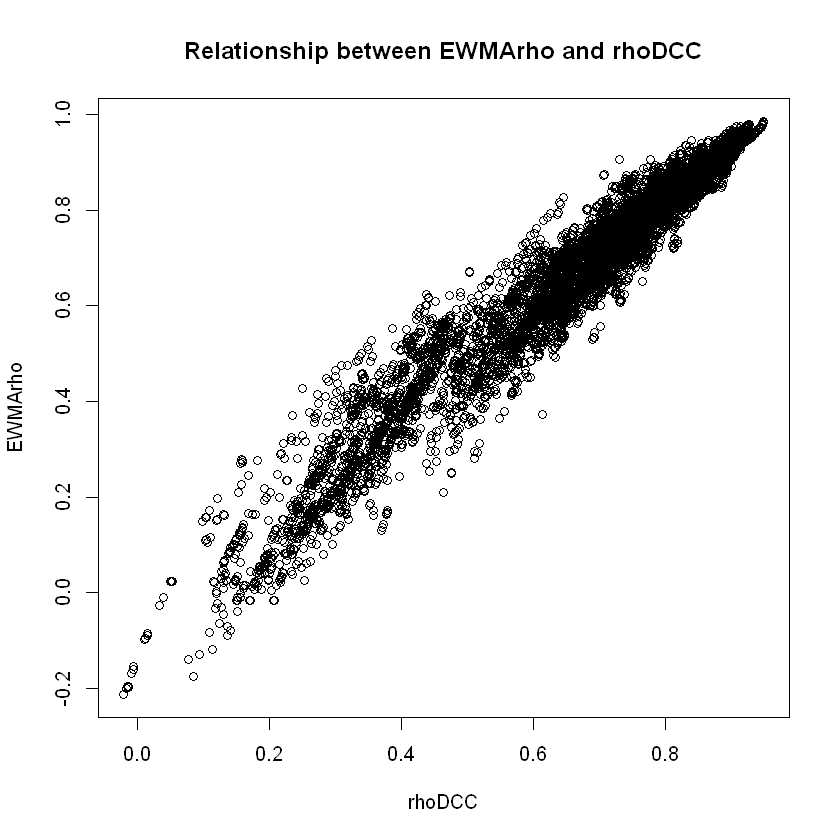Let's use colMeans() to obtain the mean of each series:

In :
# Mean of each
colMeans(cbind(EWMArho, rhoDCC, rhoDCCRich))

# Standard deviation of each
sd(EWMArho)
sd(rhoDCC)
sd(rhoDCCRich)

EWMArho
0.674422245387149
rhoDCC
0.669669105319889
rhoDCCRich
0.665214810718717
0.218757682510132
0.188780688015889
0.201356489749339

The mean of the conditional correlation for the three models is very similar. We see that the EWMA model presents the largest variability.

Now we plot only the EWMA and standard DCC models:

In :
# EWMA and standard DCC
matplot(cbind(EWMArho, rhoDCC), type = "l", lty = 1,
main = "Conditional Correlations for EWMA and DCC")
legend("bottomright", legend = c("EWMA", "DCC"), lty = 1, col = 1:2)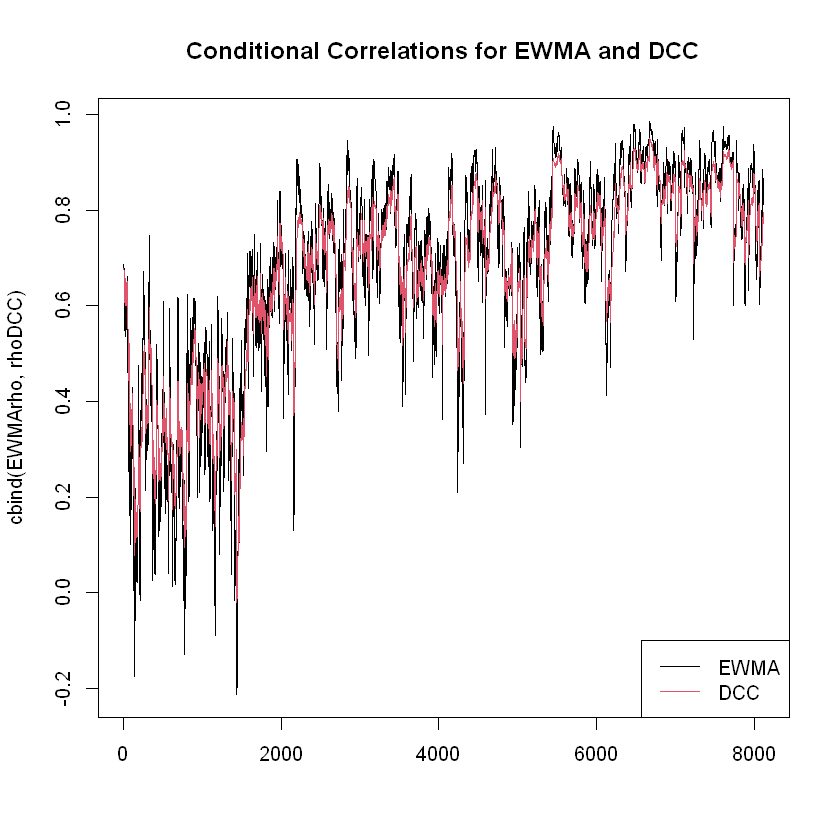## Recap¶

In this seminar we have covered:

• Multivariate volatility modelling in R
• Implementing an EWMA model
• Extracting and plotting conditional variances, covariances, and correlations for EWMA
• Fitting different specifications of DCC models
• Extracting and plotting conditional variances, covariances, and correlations for DCC
• Comparing and plotting models

Some new functions used:

• cov()
• upper.tri()
• multispec()
• dccspec()
• dccfit()

For more discussion on the material covered in this seminar, refer to Chapter 3: Multivariate volatility modeling on Financial Risk Forecasting by Jon Danielsson.

Acknowledgements: Thanks to Alvaro Aguirre and Yuyang Lin for creating these notebooks